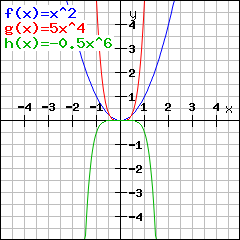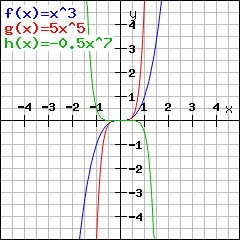# Exponentiation functionsEnter your function here. Hint: Enteras 3*x^2 ,as 3/5 andas (x+1)/(x-2x^4)

## What is a power function

A power function is a function of the form. This means it just consists of a number a and a power.

## What should I know about its symmetry?

Symmetry of power functions is simple: If the power is even, the graph of the power function is axis symmetric to the y-axis. Example:The powers are all even, therefore the power functions are axis symmetric to the y-axis.

On the other hand, all graphs of power functions with odd exponent are point symmetric to the origin:The powers are all odd, so the power functions are point symmetric to the origin.

## Why are power functions that important?

Most functions learned at school are polynomial functions. Polynomial functions are the sum of power functions.